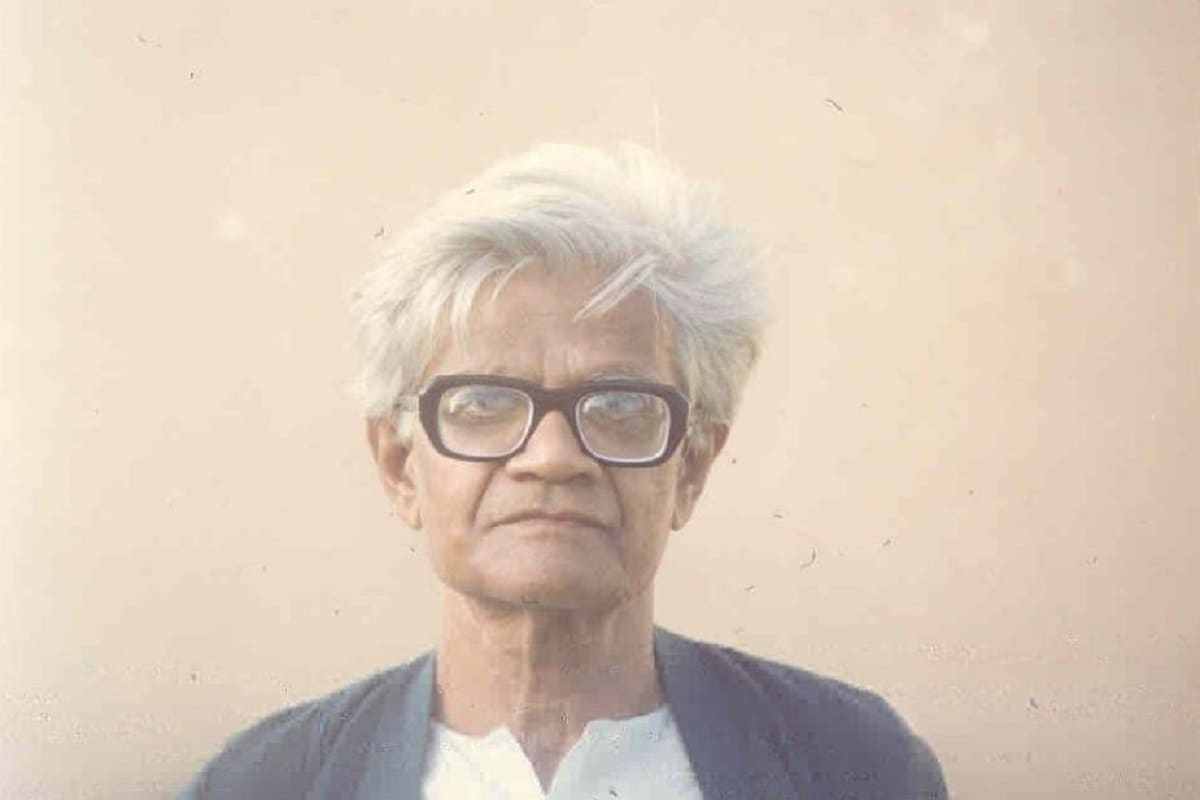#### UPSC Article

Only logged in User can create notes
Only logged in User can create notes

General Studies 3 >> Science & Technology

audio may take few seconds to load

GENERAL RELATIVITY

# GENERAL RELATIVITY

1. Context
A young Indian physicist was under pressure. His heart was set on working in the field of general relativity – the study of space, time, and gravity. But the director of his institute stood in the way. The physicist was told that he had to work on a field of the director’s choosing, or leave. He still kept at it, working on the mysteries of gravity in his spare time

2. Amal Kumar Chaudhary• Amal Kumar Raychaudhuri was an Indian physicist known for his significant contributions to general relativity and theoretical astrophysics. Born in 1923, Raychaudhuri made substantial advancements in the study of gravitational physics.
• His most famous contribution is the Raychaudhuri equation, a crucial result in general relativity that describes how neighboring trajectories of a congruence of geodesics (representing the motion of particles or light rays) in a spacetime converge or diverge under the influence of gravity.
• This equation plays a fundamental role in understanding the behavior of matter and light in gravitational fields, particularly in contexts like black hole physics and the cosmic expansion.
• Raychaudhuri's work had a lasting impact on gravitational physics and cosmology. He made important contributions to the understanding of singularities in general relativity, black hole dynamics, and the cosmic censorship hypothesis, among other areas.
• Throughout his career, Raychaudhuri received recognition for his contributions, including various awards and honors for his groundbreaking work in theoretical physics. He passed away in 2005, leaving behind a legacy that continues to influence our understanding of gravity and the cosmos
3. General Relativity• General relativity, formulated by Albert Einstein between 1907 and 1915, is a physics concept explaining gravity through the curvature of space-time. This theory unites space and time as a single entity known as space-time, attributing gravity to this curvature. Analogously, walking on a ball's surface illustrates this curvature, causing a downward curve.
• Einstein's theory relies on equations defining the connection between space-time's geometry and the energy-momentum within it. The solutions to these equations, represented by metric tensors, dictate the space-time's topology and the inertial movement of objects within it.
• However, a fundamental disparity arises between general relativity and quantum mechanics. While quantum mechanics considers time's flow as universal and fixed, general relativity interprets time's passage as flexible and relative

General relativity is described by a set of complex mathematical equations known as Einstein's field equations. These equations are a system of ten interrelated differential equations that describe how matter and energy in spacetime curve it, resulting in gravitational effects.

The full set of Einstein's field equations is quite complex, but in a simplified form, they can be written as:

Here's a brief explanation of the terms:

• represents the Einstein tensor, which describes the curvature of spacetime due to the distribution of matter and energy.
• Λ is the cosmological constant, associated with the energy density of the vacuum and the overall geometry of the universe.
• is the metric tensor, defining the geometry of spacetime.
• is the gravitational constant, determining the strength of gravity.
• is the speed of light in a vacuum.
• represents the stress-energy tensor, which describes the distribution and flow of energy and momentum in spacetime due to matter and other sources.
4. Way forward

Quantum theory and general relativity are two pillars of modern physics that describe the behavior of the universe at different scales, yet they present fundamental challenges when combined.

Quantum theory deals with the physics of the very small—particles like electrons, photons, and quarks—governing their behavior through principles like superposition, uncertainty, and wave-particle duality. It's incredibly successful in explaining phenomena at the atomic and subatomic levels.

Source: The Hindu

Share to Social